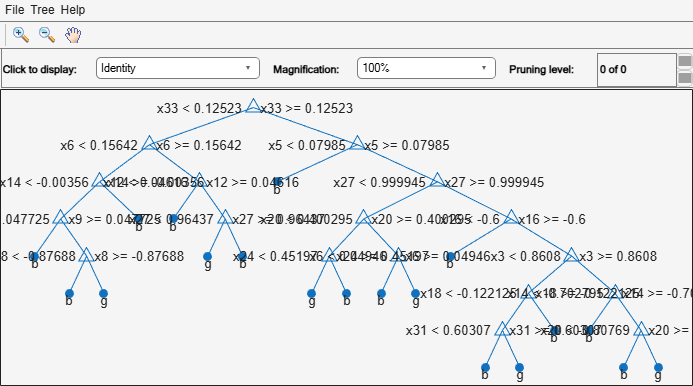# CompactTreeBagger

Compact ensemble of bagged decision trees

## Description

`CompactTreeBagger` is a compact version of the `TreeBagger` ensemble. The compact ensemble does not contain the following: information about how the `TreeBagger` function grows the decision trees; the input data used for growing trees; or the training parameters (for example, minimal leaf size, number of variables sampled for each decision split at random, and so on). Use `CompactTreeBagger` for tasks such as predicting the response or class labels.

## Creation

Create a `CompactTreeBagger` ensemble object from a full, trained `TreeBagger` ensemble by using `compact`.

## Properties

expand all

Unique class names used in the training model, specified as a cell array of character vectors.

This property is empty (`[]`) for regression trees.

Default prediction value returned by `predict`, specified as `""`, `"MostPopular"`, or a numeric scalar. This property controls the predicted value returned by the `predict` object function when no prediction is possible. You can set this property by using the `setDefaultYfit` function.

• For classification trees, you can set `DefaultYfit` to either `""` or `"MostPopular"`. If you specify `"MostPopular"` (default for classification), the property value is the name of the most probable class in the training data. If you specify `""`, the in-bag observations are excluded from computation of the out-of-bag error and margin.

• For regression trees, you can set `DefaultYfit` to any numeric scalar. The default value for regression is the mean of the response for the training data. If you set `DefaultYfit` to `NaN`, the in-bag observations are excluded from computation of the out-of-bag error and margin.

Example: ```CMdl = setDefaultYfit(CMdl,"MostPopular")```

Data Types: `single` | `double` | `char` | `string`

Split criterion contributions for each predictor, specified as a numeric vector. This property is a 1-by-Nvars vector, where Nvars is the number of changes in the split criterion. The software sums the changes in the split criterion over splits on each variable, then averages the sums across the entire ensemble of grown trees.

Data Types: `single` | `double`

Type of ensemble, specified as `"classification"` for classification ensembles or `"regression"` for regression ensembles.

Number of decision splits for each predictor, specified as a numeric vector. This property is a 1-by-Nvars vector, where Nvars is the number of predictor variables. Each element of `NumPredictorSplit` represents the number of splits on the predictor summed over all trees.

Data Types: `single` | `double`

Number of decision trees in the bagged ensemble, specified as a positive integer.

Data Types: `single` | `double`

Predictor names, specified as a cell array of character vectors. The order of the elements in `PredictorNames` corresponds to the order in which the predictor names appear in the training data `X`.

Predictive measures of variable association, specified as a numeric matrix. This property is an Nvars-by-Nvars matrix, where Nvars is the number of predictor variables. The property contains the predictive measures of variable association, averaged across the entire ensemble of grown trees.

• If you grow the ensemble with the `Surrogate` name-value argument set to `"on"`, this matrix, for each tree, is filled with the predictive measures of association averaged over the surrogate splits.

• If you grow the ensemble with the `Surrogate` name-value argument set to `"off"`, the `SurrogateAssociation` property is an identity matrix. By default, `Surrogate` is set to `"off"`.

Data Types: `single` | `double`

Decision trees in the bagged ensemble, specified as a `NumTrees`-by-1 cell array. Each tree is a `CompactClassificationTree` or `CompactRegressionTree` object.

## Object Functions

 `combine` Combine two ensembles `error` Error (misclassification probability or MSE) `margin` Classification margin `mdsprox` Multidimensional scaling of proximity matrix `meanMargin` Mean classification margin `outlierMeasure` Outlier measure for data in ensemble of decision trees `partialDependence` Compute partial dependence `plotPartialDependence` Create partial dependence plot (PDP) and individual conditional expectation (ICE) plots `predict` Predict responses using ensemble of bagged decision trees `proximity` Proximity matrix for data in ensemble of decision trees `setDefaultYfit` Set default value for `predict`

## Examples

collapse all

Reduce the size of a full ensemble of bagged classification trees by removing the training data and parameters. Then, use the compact ensemble object to make predictions on new data. Using a compact ensemble improves memory efficiency.

Load the `ionosphere` data set.

`load ionosphere`

Set the random number generator to `default` for reproducibility.

`rng("default")`

Train an ensemble of 100 bagged classification trees using the entire data set. By default, `TreeBagger` grows deep trees.

```Mdl = TreeBagger(100,X,Y,... Method="classification");```

`Mdl` is a `TreeBagger` ensemble for classification trees.

Create a compact version of `Mdl`.

`CMdl = compact(Mdl)`
```CMdl = CompactTreeBagger Ensemble with 100 bagged decision trees: Method: classification NumPredictors: 34 ClassNames: 'b' 'g' ```

`CMdl` is a `CompactTreeBagger` ensemble for classification trees.

Display the amount of memory used by each ensemble.

`whos("Mdl","CMdl")`
``` Name Size Bytes Class Attributes CMdl 1x1 993836 CompactTreeBagger Mdl 1x1 1132811 TreeBagger ```

`Mdl` takes up more space than `CMdl`.

The `CMdl.Trees` property is a 100-by-1 cell vector that contains the trained classification trees for the ensemble. Each tree is a `CompactClassificationTree` object. View the graphical display of the first trained classification tree.

`view(CMdl.Trees{1},Mode="graph");`Predict the label of the mean of `X` by using the compact ensemble.

`predMeanX = predict(CMdl,mean(X))`
```predMeanX = 1x1 cell array {'g'} ```

## Version History

Introduced in R2009a